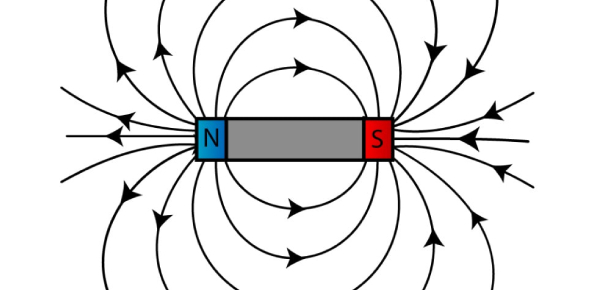# Gibilisco: Chapter 8 - Magnetism

21 Questions | Total Attempts: 513SettingsCreate your own QuizThis is the MCQs for Gibilisco: CHAPTER 8 - Magnetism

• 1.
The geomagnetic field:
• A.

Makes the earth like a huge horseshoe magnet

• B.

Runs exactly through the geographic poles

• C.

Is what makes a compass work

• D.

Is what makes an electromagnet work

• 2.
Geomagnetic lines of flux:
• A.

Are horizontal at the geomagnetic equator

• B.

Are vertical at the geomagnetic equator

• C.

Are always slanted, no matter where you go

• D.

Are exactly symmetrical around the earth, even far out into space

• 3.
A material that can be permanently magnetized is generally said to be:
• A.

Magnetic

• B.

Electromagnetic

• C.

Permanently magnetic

• D.

Ferromagnetic

• 4.
The force between a magnet and a piece of ferromagnetic metal that has not been magnetized:
• A.

Can be either repulsive or attractive

• B.

Is never repulsive

• C.

Gets smaller as the magnet gets closer to the metal

• D.

Depends on the geomagnetic field

• 5.
Magnetic flux can always be attributed to:
• A.

Ferromagnetic materials

• B.

Aligned atoms

• C.

Motion of charged particles

• D.

The geomagnetic field

• 6.
Lines of magnetic flux are said to originate:
• A.

In atoms of ferromagnetic materials

• B.

At a north magnetic pole

• C.

Where the lines converge to a point

• D.

In charge carriers

• 7.
The magnetic flux around a straight, current-carrying wire:
• A.

Gets stronger with increasing distance from the wire

• B.

Is strongest near the wire

• C.

Does not vary in strength with distance from the wire

• D.

Consists of straight lines parallel to the wire

• 8.
The gauss is a unit of:
• A.

Overall magnetic field strength

• B.

Ampere-turns

• C.

Magnetic flux density

• D.

Magnetic power

• 9.
A unit of overall magnetic field quantity is the:
• A.

Maxwell

• B.

Gauss

• C.

Tesla

• D.

Ampere-turn

• 10.
If a wire coil has 10 turns and carries 500 mA of current, what is the magnetomotive force in ampere-turns?
• A.

5000

• B.

50

• C.

5.0

• D.

0.02

• 11.
If a wire coil has 100 turns and carries 1.30 A of current, what is the magnetomotive force in gilberts?
• A.

130

• B.

76.9

• C.

164

• D.

61.0

• 12.
Which of the following is not generally possible in a geomagnetic storm?
• A.

Charged particles streaming out from the sun

• B.

Fluctuations in the earth’s magnetic field

• C.

Disruption of electrical power transmission

• D.

• 13.
An ac electromagnet:
• A.

Will attract only other magnetized objects

• B.

Will attract pure, unmagnetized iron

• C.

Will repel other magnetized objects

• D.

Will either attract or repel permanent magnets, depending on the polarity

• 14.
An advantage of an electromagnet over a permanent magnet is that:
• A.

An electromagnet can be switched on and off

• B.

An electromagnet does not have specific polarity

• C.

An electromagnet requires no power source

• D.

Permanent magnets must always be cylindrical

• 15.
A substance with high retentivity is best suited for making:
• A.

An ac electromagnet

• B.

A dc electromagnet

• C.

An electrostatic shield

• D.

A permanent magnet

• 16.
A relay is connected into a circuit so that a device gets a signal only when the relay coil carries current. The relay is probably:
• A.

An ac relay

• B.

A dc relay

• C.

Normally closed

• D.

Normally open

• 17.
A device that reverses magnetic field polarity to keep a dc motor rotating is:
• A.

A solenoid

• B.

An armature coil

• C.

A commutator

• D.

A field coil

• 18.
A high tape-recorder motor speed is generally used for:
• A.

Voices

• B.

Video

• C.

Digital data

• D.

All of the above

• 19.
An advantage of a magnetic disk, as compared with magnetic tape, for data storage and retrieval is that:
• A.

A disk lasts longer

• B.

Data can be stored and retrieved more quickly with disks than with tapes

• C.

Disks look better

• D.

Disks are less susceptible to magnetic fields

• 20.
A bubble memory is best suited for:
• A.

A large computer

• B.

A home video entertainment system

• C.

A portable cassette player

• D.

A magnetic disk

• 21.
A magnetic hard disk is usually part of
• A.

A computer

• B.

A dc motor

• C.

A tape recorder

• D.

An electromagnet

Related TopicsBack to top
×

Wait!
Here's an interesting quiz for you.